# Motion with Linear Drag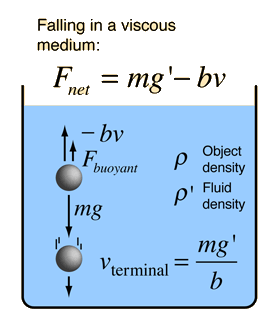### Sinking object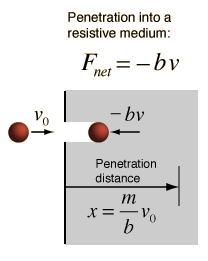### Motion equations

These are two types of problems which involve fluid friction at low velocities where it is approximately linearly dependent upon velocity.
Index

Fluid friction

 HyperPhysics***** Mechanics ***** Fluids R Nave
Go Back

# Sinking Object

For the description of an object sinking from a rest position, both the buoyant force and viscous resistance must be included.### Motion equations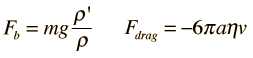Using the net gravitational accelerationyields terminal velocity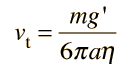and characteristic time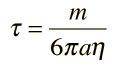.

The velocity at time t can be stated in terms of the terminal velocity and the characteristic time.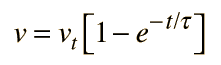The distance traveled in time t isThis motion can be modeled for a spherical object using the expression for viscous resistance in terms of the fluid viscosity.

Consider a sphere of mass m = gm = kg

and density ρ = gm/cm3 = kg/m3

corresponding to radius r = cm = m

If such a sphere sinks through a fluid of density gm/cm3 = kg/m3

the initial gravitational acceleration will be g' = m/s2 = g.

If the fluid viscosity is η = poise = ηwater

then this sinking motion will be characterized by a terminal velocity
vt = m/s

and a characteristic time τ = seconds.

If this object is released from rest, then in time t = sec,

it will fall to depth d = cm = m

and will have velocity v = cm/s = m/s

Calculation notes: You may explore variations on this problem by entering numbers and clicking outside the box to initiate the calculation. Default values will be entered for unspecified parameters, but all parameters may be changed. If you enter a mass, the radius will be calculated and vice versa. The main calculation is presumed to be that of calculating velocity and distance at a specified time, but if you enter a final velocity, a time for that velocity will be calculated. In that case you must enter a velocity less than the terminal velocity to get a physically meaningful result. It might be helpful to calculate the time associated with a specified distance, but that calculation is transcendental and must be solved numerically.

Index

Fluid friction

 HyperPhysics***** Mechanics ***** Fluids R Nave
Go Back

# Falling, Linear Drag

For the description of an object falling under the influence of a drag force which is linearly dependent upon velocity, both the buoyant force and viscous resistance must be included.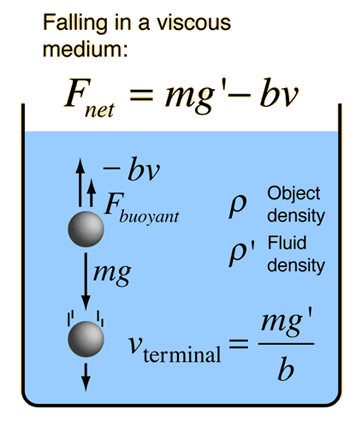### Graph of motion

A falling object will to approach a terminal velocity when the net force approaches zero.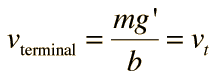For motion with initial velocity v0, the expression for velocity becomesand expressed in terms of the terminal velocity vt and the characteristic time τ = m/b , it takes the form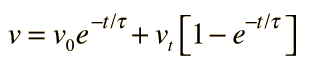.

The distance traveled in time t is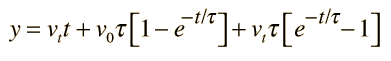.
Index

Fluid friction

 HyperPhysics***** Mechanics ***** Fluids R Nave
Go Back